righto.com Go back Open original

# Two bits per transistor: high-density ROM in Intel's 8087 floating point chip

The 8087 chip provided fast floating point arithmetic for the original IBM PC and became part of the x86 architecture used today. One unusual feature of the 8087 is it contained a multi-level ROM (Read-Only Memory) that stored two bits per transistor, twice as dense as a normal ROM. Instead of storing binary data, each cell in the 8087's ROM stored one of four different values, which were then decoded into two bits. Because the 8087 required a large ROM for microcode1 and the chip was pushing the limits of how many transistors could fit on a chip, Intel used this special technique to make the ROM fit. In this article, I explain how Intel implemented this multi-level ROM.

Intel introduced the 8087 chip in 1980 to improve floating-point performance on the 8086 and 8088 processors. Since early microprocessors operated only on integers, arithmetic with floating point numbers was slow and transcendental operations such as trig or logarithms were even worse. Adding the 8087 co-processor chip to a system made floating point operations up to 100 times faster. The 8087's architecture became part of later Intel processors, and the 8087's instructions (although now obsolete) are still a part of today's x86 desktop computers.

I opened up an 8087 chip and took die photos with a microscope yielding the composite photo below. The labels show the main functional blocks, based on my reverse engineering. (Click here for a larger image.) The die of the 8087 is complex, with 40,000 transistors.2 Internally, the 8087 uses 80-bit floating point numbers with a 64-bit fraction (also called significand or mantissa), a 15-bit exponent and a sign bit. (For a base-10 analogy, in the number 6.02x1023, 6.02 is the fraction and 23 is the exponent.) At the bottom of the die, "fraction processing" indicates the circuitry for the fraction: from left to right, this includes storage of constants, a 64-bit shifter, the 64-bit adder/subtracter, and the register stack. Above this is the circuitry to process the exponent.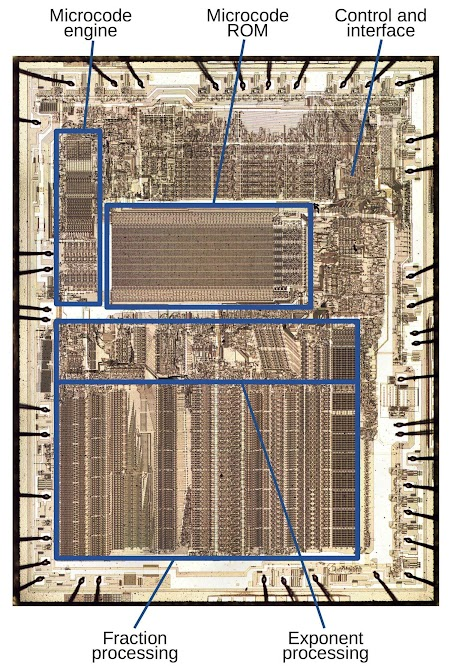Die of the Intel 8087 floating point unit chip, with main functional blocks labeled.

An 8087 instruction required multiple steps, over 1000 in some cases. The 8087 used microcode to specify the low-level operations at each step: the shifts, adds, memory fetches, reads of constants, and so forth. You can think of microcode as a simple program, written in micro-instructions, where each micro-instruction generated control signals for the different components of the chip. In the die photo above, you can see the ROM that holds the 8087's microcode program. The ROM takes up a large fraction of the chip, showing why the compact multi-level ROM was necessary. To the left of the ROM is the "engine" that ran the microcode program, essentially a simple CPU.

The 8087 operated as a co-processor with the 8086 processor. When the 8086 encountered a special floating point instruction, the processor ignored it and let the 8087 execute the instruction in parallel.3 I won't explain in detail how the 8087 works internally, but as an overview, floating point operations were implemented using integer adds/subtracts and shifts. To add or subtract two floating point numbers, the 8087 shifted the numbers until the binary points (i.e. the decimal points but in binary) lined up, and then added or subtracted the fraction. Multiplication, division, and square root were performed through repeated shifts and adds or subtracts. Transcendental operations (tan, arctan, log, power) used CORDIC algorithms, which use shifts and adds of special constants, processing one bit at a time. The 8087 also dealt with many special cases: infinities, overflows, NaN (not a number), denormalized numbers, and several rounding modes. The microcode stored in ROM controlled all these operations.

## Implementation of a ROM

The 8087 chip consists of a tiny silicon die, with regions of the silicon doped with impurities to give them the desired semiconductor properties. On top of the silicon, polysilicon (a special type of silicon) formed wires and transistors. Finally, a metal layer on top wired the circuitry together. In the photo below, the left side shows a small part of the chip as it appears under a microscope, magnifying the yellowish metal wiring. On the right, the metal has been removed with acid, revealing the polysilicon and silicon. When polysilicon crosses silicon, a transistor is formed. The pink regions are doped silicon, and the thin vertical lines are the polysilicon. The small circles are contacts between the silicon and metal layers, connecting them together.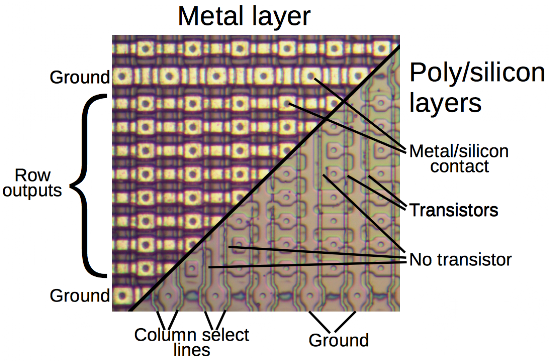Structure of the ROM in the Intel 8087 FPU. The metal layer is on the left and the polysilicon and silicon layers are on the right.

While there are many ways of building a ROM, a typical way is to have a grid of "cells," with each cell holding a bit. Each cell can have a transistor for a 0 bit, or lack a transistor for a 1 bit. In the diagram above, you can see the grid of cells with transistors (where silicon is present under the polysilicon) and missing transistors (where there are gaps in the silicon). To read from the ROM, one column select line is energized (based on the address) to select the bits stored in that column, yielding one output bit from each row. You can see the vertical polysilicon column select lines and the horizontal metal row outputs in the diagram. The vertical doped silicon lines are connected to ground.

The schematic below (corresponding to a 4×4 ROM segment) shows how the ROM functions. Each cell either has a transistor (black) or no transistor (grayed-out). When a polysilicon column select line is energized, the transistors in that column turn on and pull the corresponding metal row outputs to ground. (For our purposes, an NMOS transistor is like a switch that is open if the input (gate) is 0 and closed if the input is 1.) The row lines output the data stored in the selected column.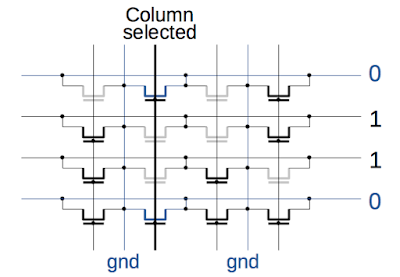Schematic of a 4×4 segment of a ROM.

The column select signals are generated by a decoder circuit. Since this circuit is built from NOR gates, I'll first explain the construction of a NOR gate. The schematic below shows a four-input NOR gate built from four transistors and a pull-up resistor (actually a special transistor). On the left, all inputs are 0 so all the transistors are off and the pull-up resistor pulls the output high. On the right, an input is 1, turning on a transistor. The transistor is connected to ground, so it pulls the output low. In summary, if any inputs are high, the output is low so this circuit implements a NOR gate.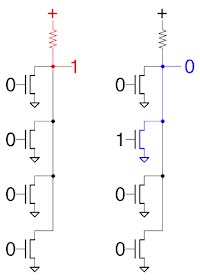4-input NOR gate constructed from NMOS transistors.

The column select decoder circuit takes the incoming address bits and activates the appropriate select line. The decoder contains an 8-input NOR gate for each column, with one NOR gate selected for the desired address. The photo shows two of the NOR gates generating two of the column select signals. (For simplicity, I only show four of the 8 inputs). Each column uses a different combination of address lines and complemented address lines as inputs, selecting a different address. The address lines are in the metal layer, which was removed for the photo below; the address lines are drawn in green. To determine the address associated with a column, look at the square contacts associated with each transistor and note which address lines are connected. If all the address lines connected to a column's transistors are low, the NOR gate will select the column.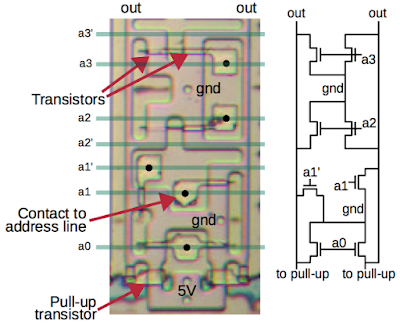Part of the address decoder. The address decoder selects odd columns in the ROM, counting right to left. The numbers at the top show the address associated with each output.

The photo below shows a small part of the ROM's decoder with all 8 inputs to the NOR gates. You can read out the binary addresses by carefully examining the address line connections. Note the binary pattern: a1 connections alternate every column, a2 connections alternate every two columns, a3 connections every four columns, and so forth. The a0 connection is fixed because this decoder circuit selects the odd columns; a similar circuit above the ROM selects the even addresses. (This split was necessary to make the decoder fit on the chip because each decoder column is twice as wide as a ROM cell.)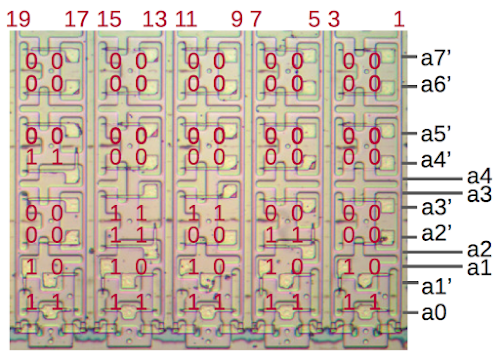Part of the address decoder for the 8087's microcode ROM. The decoder converts an 8-bit address into column select signals.

The last component of the ROM is the set of multiplexers that reduces the 64 output rows down to 8 rows.4 Each 8-to-1 multiplexer selects one of its 8 inputs, based on the address. The diagram below shows one of these row multiplexers in the 8087, built from eight large pass transistors, each one connected to one of the row lines. All the transistors are connected to the output so when the selected transistor is turned on, it passes its input to the output. The multiplexer transistors are much, much larger than the transistors in the ROM to reduce distortion of the ROM signal. A decoder (similar to the one discussed earlier, but smaller) generates the eight multiplexer control lines from three address lines.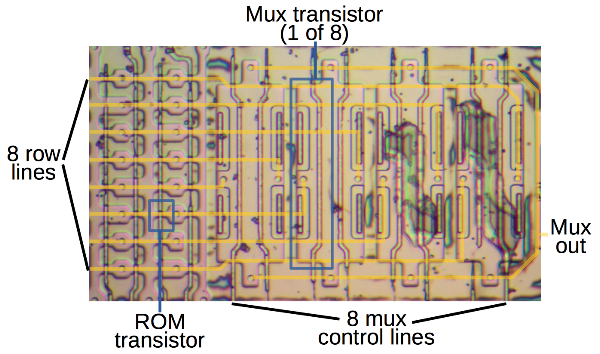One of eight row multiplexers in the ROM. This shows the poly/silicon layers, with metal wiring drawn in orange.

To summarize, the ROM stores bits in a grid. It uses eight address bits to select a column in the grid. Then three address bits select the desired eight outputs from the row lines.

## The multi-level ROM

The discussion so far explained of a typical ROM that stores one bit per cell. So how did 8087 store two bits per cell? If you look closely, the 8087's microcode ROM has four different transistor sizes (if you count "no transistor" as a size).6 With four possibilities for each transistor, a cell can encode two bits, approximately doubling the density.7 This section explains how the four transistor sizes generate four different currents, and how the chip's analog and digital circuitry converts these currents into two bits.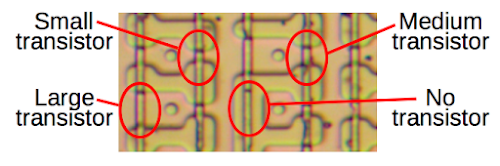A closeup of the 8087's microcode ROM shows four different transistor sizes. This allows the ROM to store two bits per cell.

The size of the transistor controls the current through the transistor.8 The important geometric factor is the varying width of the silicon (pink) where it is crossed by the polysilicon (vertical lines), creating transistors with different gate widths. Since the gate width controls the current through the transistor, the four transistor sizes generate four different currents: the largest transistor passes the most current and no current will flow if there is no transistor at all.

The ROM current is converted to bits in several steps. First, a pull-up resistor converts the current to a voltage. Next, three comparators compare the voltage with reference voltages to generate digital signals indicating if the ROM voltage is lower or higher. Finally, logic gates convert the comparator output signals to the two output bits. This circuitry is repeated eight times, generating 16 output bits in total.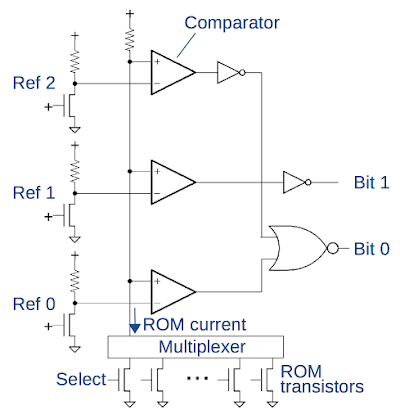The circuit to read two bits from a ROM cell.

The circuit above performs these conversion steps. At the bottom, one of the ROM transistors is selected by the column select line and the multiplexer (discussed earlier), generating one of four currents. Next, a pull-up resistor12 converts the transistor's current to a voltage, resulting in a voltage depending on the size of the selected transistor. The comparators compare this voltage to three reference voltages, outputting a 1 if the ROM voltage is higher than the reference voltage. The comparators and reference voltages require careful design because the ROM voltages could differ by as little as 200 mV.

The reference voltages are mid-way between the expected ROM voltages, allowing some fluctuation in the voltages. The lowest ROM voltage is lower than all the reference voltages so all comparators will output 0. The second ROM voltage is higher than Reference 0, so the bottom comparator outputs 1. For the third ROM voltage, the bottom two comparators output 1, and for the highest ROM voltage all comparators output 1. Thus, the three comparators yield four different output patterns depending on the ROM transistor. The logic gates then convert the comparator outputs into the two output bits.10

The design of the comparator is interesting because it is the bridge between the analog and digital worlds, producing a 1 or 0 if the ROM voltage is higher or lower than the reference voltage. Each comparator contains a differential amplifier that amplifies the difference between the ROM voltage and the reference voltage. The output from the differential amplifier drives a latch that stabilizes the output and converts it to a logic-level signal. The differential amplifier (below) is a standard analog circuit. A current sink (symbol at the bottom) provides a constant current. If one of the transistors has a higher input voltage than the other, most of the current passes through that transistor. The voltage drop across the resistors will cause the corresponding output to go lower and the other output to go higher.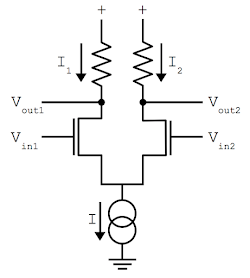Diagram showing the operation of a differential pair. Most of the current will flow through the transistor with the higher input voltage, pulling the corresponding output lower. The double-circle symbol at the bottom is a current sink, providing a constant current I.

The photo below shows one of the comparators on the chip; the metal layer is on top, with the transistors underneath. I'll just discuss the highlights of this complex circuit; see the footnote12 for details. The signal from the ROM and multiplexer enters on the left. The pull-up circuit12 converts the current into a voltage. The two large transistors of the differential amplifier compare the ROM's voltage with the reference voltage (entering at top). The outputs from the differential amplifier go to the latch circuitry (spread across the photo); the latch's output is in the lower right. The differential amplifier's current source and pull-up resistors are implemented with depletion-mode transistors. Each output circuit uses three comparators, yielding 24 comparators in total.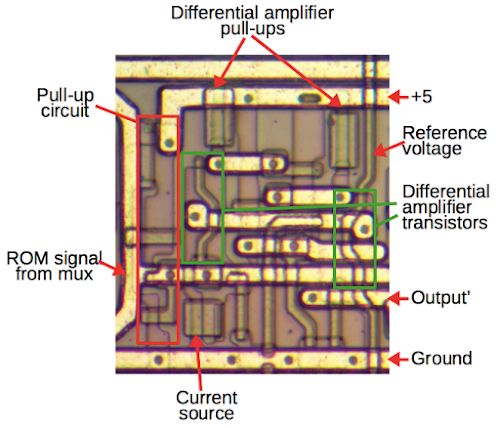One of the comparators in the 8087. The chip contains 24 comparators to convert the voltage levels from the multi-level ROM into binary data.

Each reference voltage is generated by a carefully-sized transistor and a pull-up circuit. The reference voltage circuit is designed as similar as possible to the ROM's signal circuitry, so any manufacturing variations in the chip will affect both equally. The reference voltage and ROM signal both use the same pull-up circuit. In addition, each reference voltage circuit includes a very large transistor identical to the multiplexer transistor, even though there is no multiplexing in the reference circuit, just to make the circuits match. The three reference voltage circuits are identical except for the size of the reference transistor.9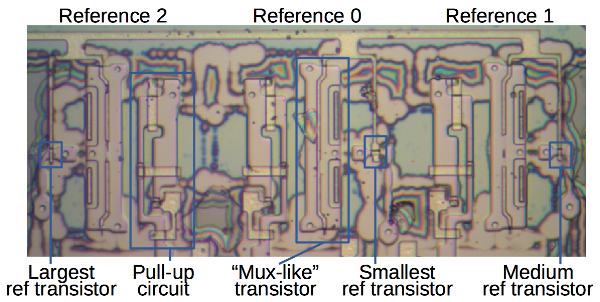Circuit generating the three reference voltages. The reference transistors are sized between the ROM's transistor sizes. The oxide layer wasn't fully removed from this part of the die, causing the color swirls in the photo.

Putting all the pieces together, the photo below shows the layout of the microcode ROM components on the chip.12 The bulk of the ROM circuitry is the transistors holding the data. The column decoder circuitry is above and below this. (Half the column select decoders are at the top and half are at the bottom so they fit better.) The output circuitry is on the right. The eight multiplexers reduce the 64 row lines down to eight. The eight rows then go into the comparators, generating the 16 output bits from the ROM at the right. The reference circuit above the comparators generates the three reference voltage. At the bottom right, the small row decoder controls the multiplexers.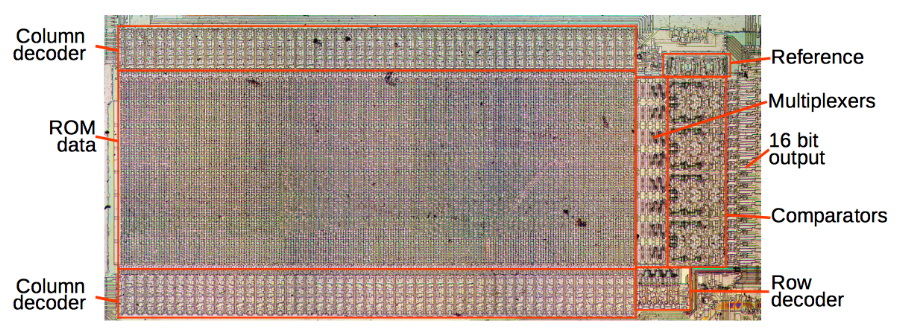Microcode ROM from the Intel 8087 FPU with main components labeled.

While you'd hope for the multi-level ROM to be half the size of a regular ROM, it isn't quite that efficient because of the extra circuitry for the comparators and because the transistors were slightly larger to accommodate the multiple sizes. Even so, the multi-level ROM saved about 40% of the space a regular ROM would have taken.

Now that I have determined the structure of the ROM, I could read out the contents of the ROM simply (but tediously) by looking at the size of each transistor under a microscope. But without knowing the microcode instruction set, the ROM contents aren't useful.

## Conclusions

The 8087 floating point chip used an interesting two-bit-per-cell structure to fit the microcode onto the chip. Intel re-used the multi-level ROM structure in 1981 in the doomed iAPX 432 system.11 As far as I can tell, interest in ROMs with multiple-level cells peaked in the 1980s and then died out, probably because Moore's law made it easier to gain ROM capacity by shrinking a standard ROM cell rather than designing non-standard ROMs requiring special analog circuits built to high tolerances.14

Surprisingly, the multi-level concept has recently returned, but this time in flash memory. Many flash memories store two or more bits per cell.13 Flash has even achieved a remarkable 4 bits per cell (requiring 16 different voltage levels) with "quad-level cell" consumer products announced recently. Thus, an obscure technology from the 1980s can show up again decades later.

I announce my latest blog posts on Twitter, so follow me at @kenshirriff for future 8087 articles. I also have an RSS feed.